Mobile QR Code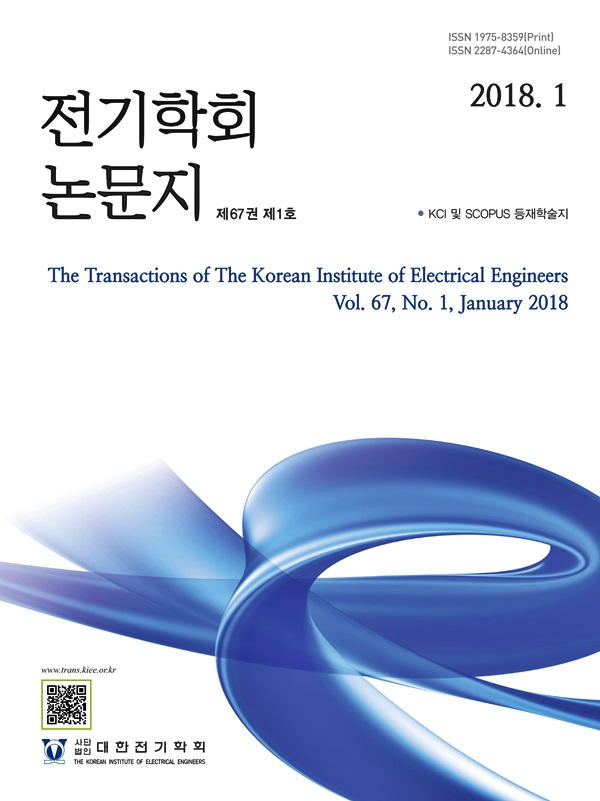The Transactions of the Korean Institute of Electrical Engineers

ISO Journal TitleTrans. Korean. Inst. Elect. Eng.

1. (Dept. of Transportation Engineering, Korea University of Science and Technology, Korea.)
2. (Smart Electrical & Signaling Division, Korea Railroad Research Institute, Korea.)

Energy storage system, Contribution analysis, DC Railroad, Fault Selective Device, Fault characteristic

1. Introduction

In order to respond to the rapid climate change around the world, many countries in including Korea, designated and implemented voluntary greenhouse gas reduction targets. In the field of power system, many studies on carbon reduction are in progress by reducing the use of fossil fuels by using Energy Storage System (ESS) or renewable energy. In case of Korea, various national policies such as mandatory installation of ESS by public institutions are putting an effort to respond to climate change(1-3).

The rail transport sector in Korea is a public institution, but it is not included in the mandatory ESS installation list. In consideration of policy and institutional changes, various specific studies on the use of ESS in the rail transport sector are also needed. Also, in previous studies, many studies have been conducted on the optimal capacity calculation or operation optimization through ESS linkage with DC electric railways, but no studies have been conducted on whether the existing protection system operates.(4-7).

Among electric railroads, urban railroads are mainly operated in downtown areas, have short distances between stations, are mostly used as direct current, and have high load usage during rush hours. Because of these characteristics, when used in conjunction with ESS, its utility is high, such as reducing peak load and utilizing train's regenerative braking energy. However, when an accident such as a short circuit occurs while ESS is connected, as the system becomes more complicated, there is a possibility that the existing relay may malfunction.

Therefore, in this paper, the accident characteristics according to the ESS connection were analyzed according to the ESS operation state, the presence or absence of failure resistance, and the train operating conditions. In addition, it was analyzed how it affects the setting of Fault Selective Device(50F) in current protection system in DC railroad substation. For transient analysis, DC electric rail system and accidents were simulated using the power analysis program PSCAD/EMTDC.

2. Conventional Protection method in DC Railroad

The protective relay elements mainly used in the DC power supply system of urban railroads are the DC Over current Relay (76I), in which the circuit breaker operates directly to protect the power supply system, and the Fault Selective Device(50F) that detects faults by distinguishing the maximum traction current and the short-circuit fault current.

2.1 DC Over current Relay(76I)

The DC over current relay is used to prevent the energization when the current exceeding the rated current continues for a certain period of time, as shown in Figure 1.

그림. 1. DC 과전류 계전기 회로도

Fig. 1. Circuit diagram of DC over current relay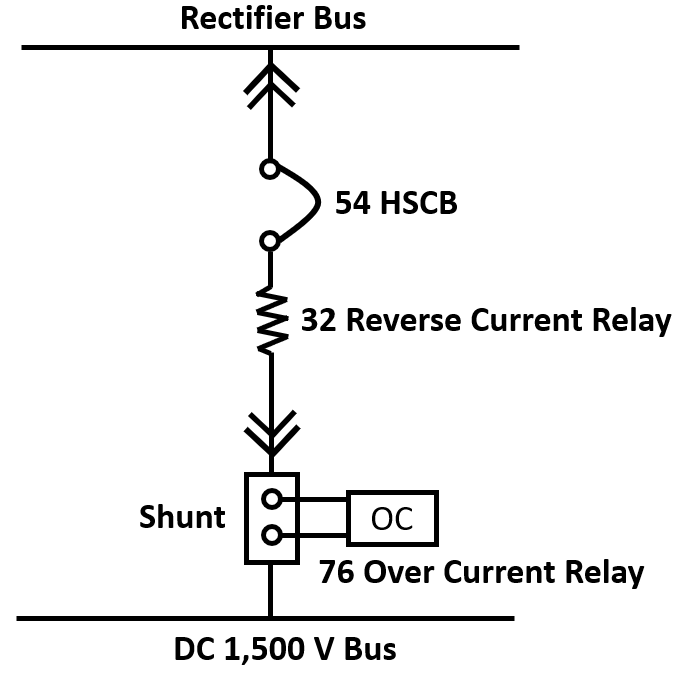The DC over current relay is installed between the positive pole busbar on the positive pole side of the rectifier and on each feeder side branched from the positive pole busbar. When an over current flows, a circuit breaker is used to protect the power supply circuit(8).

2.2 Fault Selective Device(50F)

The fault selective device classifies the characteristics of fault currents such as general load current and short circuit using the current changing rate (di/dt) method and the differential current (ΔI). The load current characteristics of the electric railway go through several small changes in the current width until the maximum value of the load current is reached.

The following relationship is established between the maximum value of $\triangle I_{L}$ and the maximum value of the feeding current.

(1)
$\triangle I_{\max}=I_{L,\:\max}\times(0.3\sim 0.5)$

Unlike general load currents, in the case of a fault current, a current is generated differently depending on a fault caused by a short circuit or an arc, the location of the fault, etc.

그림. 2. DC 전기철도의 a)부하전류와 b)고장전류 비교

Fig. 2. Comparison of a) Load current and b) fault current of DC electric railroad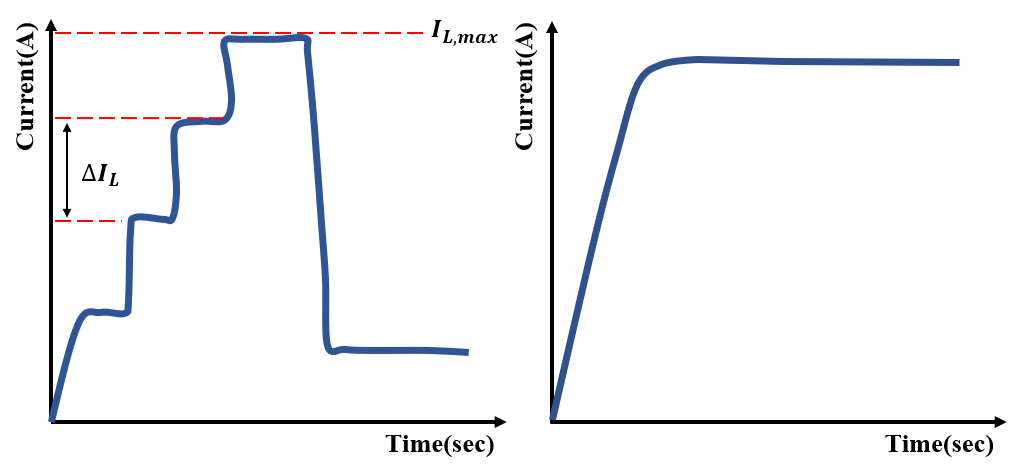A fault occurring in a DC power supply circuit has a high slope and appears in the form of a large current, so faults can be detected through the change in slope and current. However, train load’s starting current has a shape very similar to the fault current, so it is difficult to identify the fault. In addition, when a fault occurs at a location far away from the relay, it is difficult to detect a fault because the slope is low and the amount of change in current is not large, unlike a nearby fault. Therefore, the fault selection device uses both the current change rate method and the differential current method.

2.2.1 $\triangle I$ method

When the rate of change of current exceeds a certain slope E, $\triangle I$ calculation starts. If $\triangle I$ is less than $\triangle I_{\max}$, which is setting value of that system, relay does not trip. When it becomes larger, it operated in two ways depending on the situation(9).

First, if the current is maintained for $t_{\triangle I}$ and the current change rate is greater than F, which is end slope, the relay is tripped.

If di/dt is less than F, which is the end slope for $t_{rst}$, reset the relay. The algorithm for the differential current method is shown in Figure 3.

그림. 3. △I 보호방식 알고리즘

Fig. 3. △I protection algorithm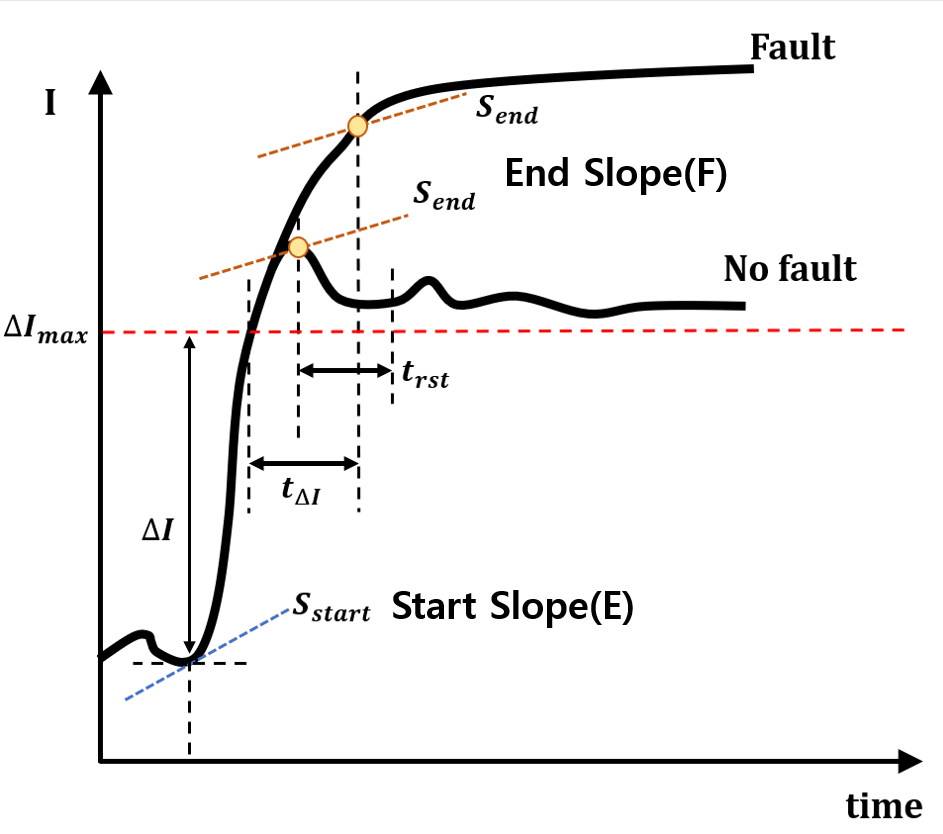2.2.2 di/dt method

If di/dt exceeds the starting slope E, then time counting and $\triangle I$ calculation starts. If time counting exceeds $t_{di/dt}$, it works in two ways. If $\triangle I$ is greater than the system setting value $I_{\min}$, and di/dt is greater than the slope of E, the relay trips. If di/dt is less than F, reset the relay.

그림. 4. di/dt 보호방식 알고리즘

Fig. 4. di/dt protection algorithm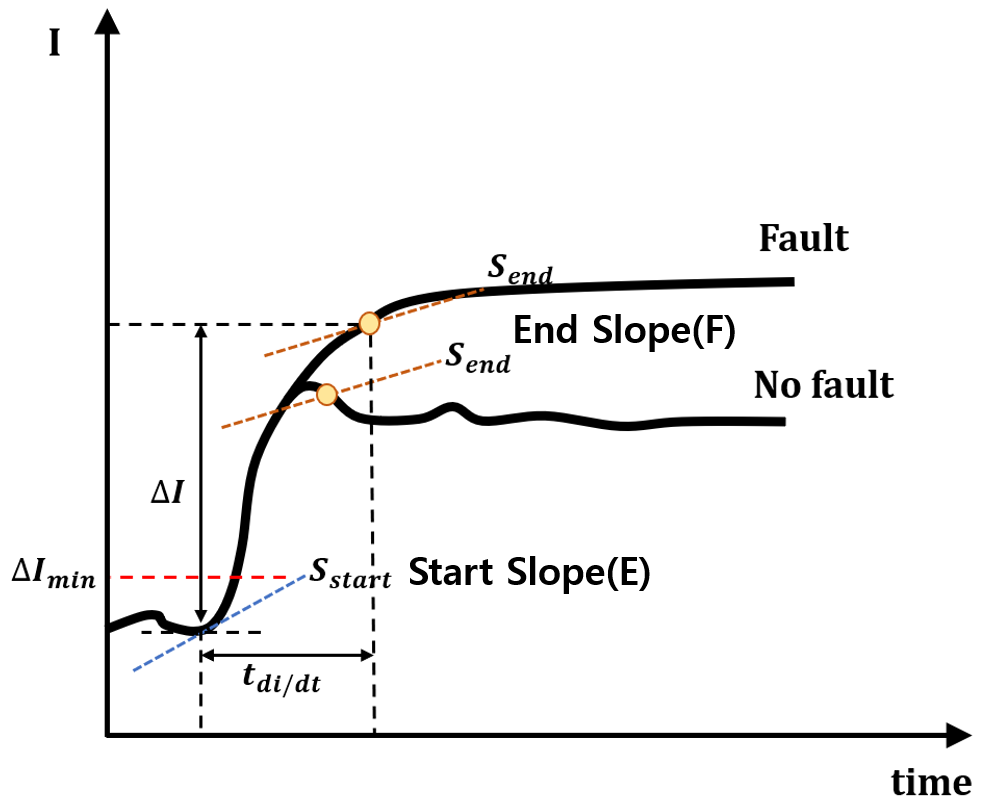In this way, when fault determination is made by comparing the current gradient with the current difference, an additional disturbance such as an ESS will affect the current change, so a contribution analysis is required(9).

3. ESS Connection Method to DC Railroad

ESS has advantages and disadvantages depending on the location where it is installed, such as a substation or a catenary. In this paper, three types of linkage methods are introduced(10).

When connected to DC-link, the battery that uses DC is connected only by transforming it using a DC/DC converter, so the loss is small from the point of view of energy conversion efficiency. However, if a large-capacity battery is connected, the contribution to the system increases when a disturbance occurs, which adversely affects stability.

3.2 Stand-alone Type Catenary Connection

In the case of direct connection to the catenary, like the DC-link connection method, it shows high efficiency in terms of power conversion efficiency and increases energy storage efficiency for regenerative braking of trains. However, the ESS linkage adversely affects the voltage stability of the catenary.

3.3 AC Bus Connection

Unlike the previous methods, the method is linked to the power receiving point supplied by the power supplier. Since ESS is linked to alternating current, the energy conversion efficiency is low and it is difficult to utilize the regenerative braking energy of the train.

In this paper, a DC-link linkage method with advantages of power conversion efficiency and stability and regenerative braking energy utilization is selected, and a summary of the three methods is shown in Table 1(11).

표 1. 연결방식별 비교

Table 1. Comparison of features by connection method

 Connection Method No. of power conversion(s) from ESS to load Possibility of linking renewable energy Utilizing regenerative braking energy DC-link Bus 1 High Possible with low efficiency Stand-alone 1 Low possible AC Bus 4 Very High impossible

4. Simulation Results

In this paper, when the ESS in the substation of the DC electric railroad is connected to the DC-link terminal, the situation when a fault occurs in the catenary is simulated. First, the operation of the ESS is determined according to the magnitude of the voltage $V_{ess}$ of the connection point where the ESS is connected to the main power source. When the load current is maximum, a voltage drop occurs, and when the current is supplied to the ESS through regenerative braking of the train, charging is performed and the voltage rises. According to the international standard IEC 60850, the minimum non-permanent voltage is 1.0 kV based on DC 1.5 kV, and the highest non-permanent voltage is DC 1.95 kV(12). Therefore, in this paper, discharging of the ESS is indicated when $V_{ess}$ falls below 1.1 kV, and charging is indicated when $V_{ess}$ is 1.7 kV.

The capacity of the connected ESS differs depending on the system conditions, and the capacity was calculated by considering the charging and discharging states, focusing on the amount of regenerative energy generated from the train. Since the purpose of this paper is to analyze the contribution of the ESS when an accident occurs during charging or discharging, modeling of the ESS and the bidirectional converter uses current source-based average modeling to indicate that current is supplied.

In this paper, the maximum load current state (ESS discharge) and the ESS charging state were simulated and compared with the slope of the fault current in the no-load state. The slope at which the failure selection device judges the failure is assumed to be Start Slope = 50 kA/s and End Slope = 15 kA/s, which are used as representative values. The $t_{di/dt}$ value is determined dependently according to the conditions of the system. In general, in 1,500 V DC electric railways, $t_{di/dt}$ is set to around 40 ms, and in this paper, $t_{di/dt}$ is set to 40 ms.

The magnitude of the fault resistance was assumed to be 50 mΩ when the arc occurred. The train operating conditions were classified into two types: a condition in which no train is operated (R=100 kΩ) and a condition in which train operation is maximum (R=0.474 Ω). Since the distance between substations of DC electric railway is about 2~4 km, in this paper, it is assumed that the failure occurred at 2 km, which is the middle point. Other parameters required for simulation are shown in Table 2.

표 2. 시스템 파라미터

Table 2. System parameters

 Sorts Set value Catenary Resistance 12.8 mΩ/km Rail Resistance 14 mΩ/km Track Inductance 1.0 mH/km Fault occurring time 3 s Duration of fault 1 s

Figure 5 shows the circuit diagram of the DC electric railway and the modeling circuit diagram in which the ESS is connected to the substation using PSCAD/EMTDC. It is assumed that the ESS is connected to the DC-link stage through the rectifier transformer and rectifier from the main power source, the train is moving on the upward direction at 2 km, and the fault location occurred near the train.

그림. 5. PSCAD로 나타낸 직류 전기철도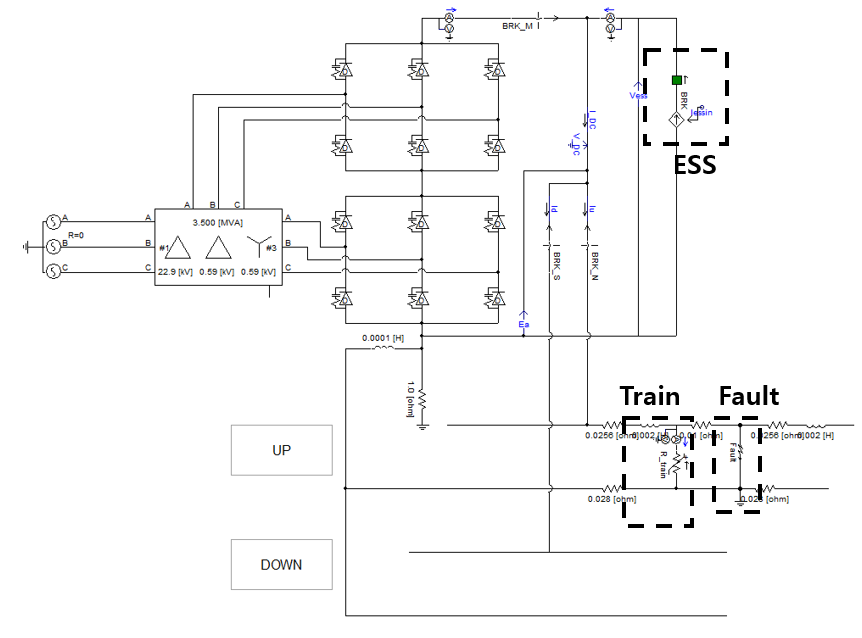Before the simulation analysis, the terms are explained as follows.

$V_{L}$ : Load voltage

$V_{DC}$ : Rectifier voltage(=$V_{ESS}$)

$I_{ess}$ : ESS charging and discharging current

$I_{s}$ : Source current

$I_{DC}$ : Rectifier current

$I_{l}$ : Load current

In case of no load condition, the no load voltage before the accident was 1.6 kV, and the voltage after the failure was reduced to 0.432 kV.

그림. 6. 무부하 상태 a) 전압 b) 전류 c) di/dt

Fig. 6. No load condition a) voltage b) current c) di/dt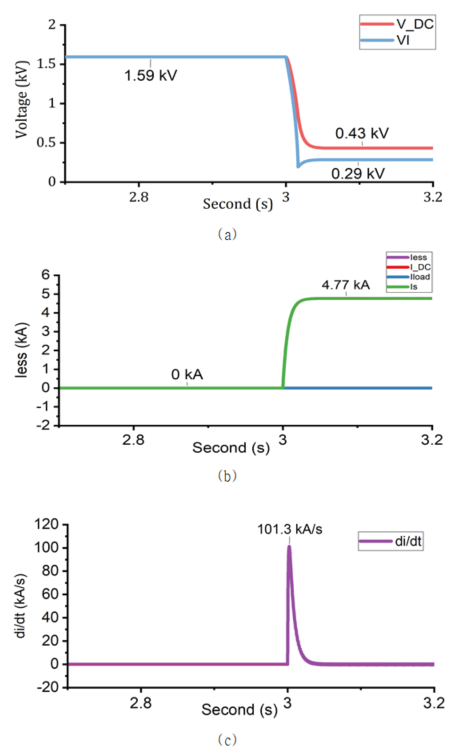Since there is no load, the current flowing through the relay increased from 0 to 4.769 kA, and the slope was 101.3 kA/s, confirming the trip of the 50F relay.

4.2 ESS charging condition

This condition occurs when the regenerative energy generated while the train brakes, which causes the flow of current from train to ESS.

그림. 7. ESS 충전 상태 a) 전압 b) 전류 c) di/dt

Fig. 7. ESS charging condition a) voltage b) current c) di/dt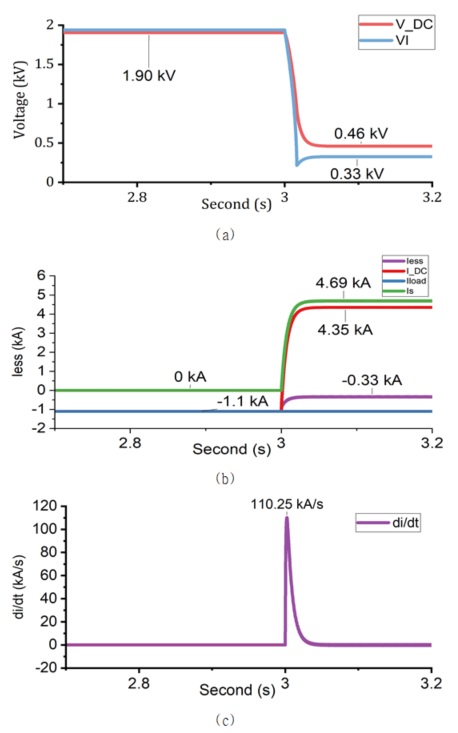In the case of the regenerative braking state, the current flowing to the ESS is generated, and when a fault occurs, the slope has a higher slope value than the no-load condition, and the relay trip was confirmed.

When the maximum load current flows (load resistance = 0.474Ω), it drops below 1.1 kV and the ESS performs a discharge operation. First, Figure 8 shows the state of the maximum load current before the ESS is connected.

그림. 8. 최대부하전류 상태(ESS 미연계) a) 전압 b) 전류 c) di/dt

Fig. 8. Maximum load current condition without ESS a) voltage b) current c) di/dt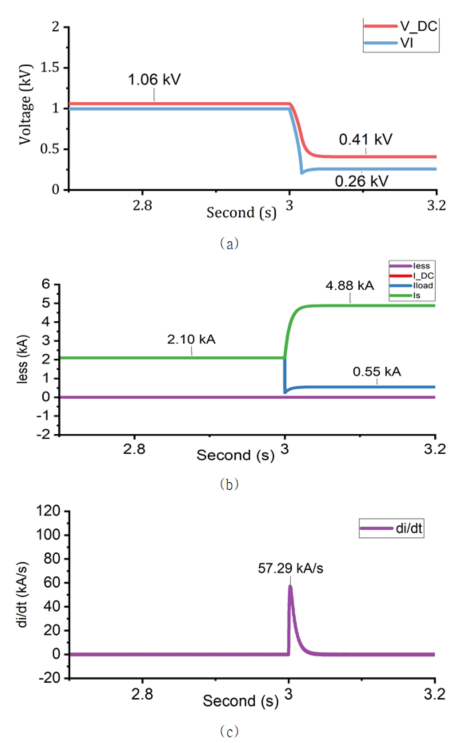When a short-circuit failure occurred while the maximum load current was generated, the current change slope was relatively lower than that of the no-load condition or the ESS charged condition due to the influence of the load current, and a value of 57.29 kA/s was derived.

When the ESS is connected in the state of the maximum load current, the voltage $V_{ess}$ rises due to the discharge operation of the ESS.

Figure 9 shows full load condition while ESS is in operation.

그림. 9. 최대부하전류 상태(ESS 연계) a) 전압 b) 전류 c) di/dt

Fig. 9. Maximum load current condition with ESS a) voltage b) current c) di/dt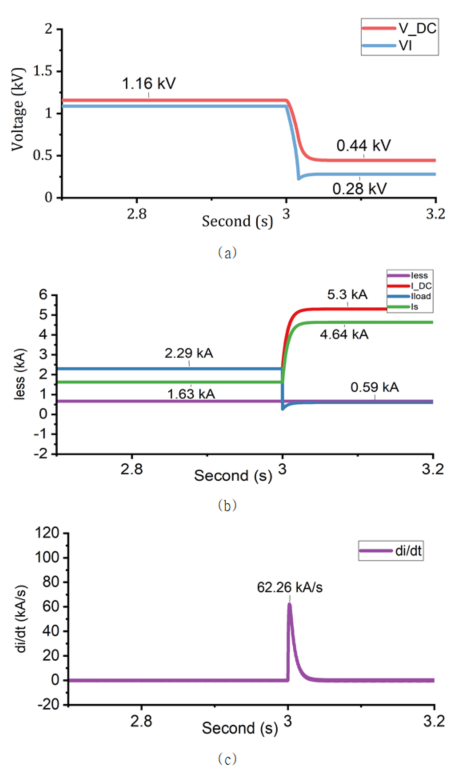Due to the increase of the voltage while connecting to the ESS, the current change occurred more significantly, and 62.26 kA/s was derived. It was confirmed that the relay responds more sensitively because of ESS. If current was originally supplied from the main power source to the load, the situation in which the ESS connected helps the main power source to supply the current to the load. Therefore, when the feeder voltage is increased and the current is changed accordingly, a larger change occurs. Table 3 shows the result values ​​derived earlier. Since the location where the fault occurred was not far from the relay, the line inductance was small and magnitude of the current was high. Therefore, most of the faults were detected by $\triangle I$.

표 3. 케이스별 di/dt 결괏값

Table 3. di/dt results by cases

 Condition di/dt(kA/s) $I_{DC}$ before the fault(kA) $I_{DC}$ after the fault(kA) No load 101.3 0 4.77 ESS charging 110.25 -1.1 4.35 max. load current w/o ESS 57.29 2.1 4.88 max. load current w/ ESS 62.26 2.29 5.30

5. Conclusion

In this paper, change of di/dt characteristic was analyzed by changing the presence of failure resistance, ESS operation condition, and train operating condition. As a result of simulating the DC electric rail system using the power analysis program PSCAD/ EMTDC and analyzing the slope of the 50F relay for each case, the existing system tripped sufficiently even after ESS connection. However, since the voltage rises according to the use of the ESS, the change in current is larger when a failure occurs, resulting in a higher slope. As a result, it is necessary to pay attention to the change in slope sensitivity when using the ESS.

Acknowledgements

This research was supported by a grant from the R&D program of the Korea Railroad Research Institute, Republic of Korea.

References

1
South Korean Ministry of Trade, Industry and Energy, , 5th Renewable Energy Technology Development and Dissemination Basic Plan, Dec. 2020.2
Korea Energy Agency, August 2020, ESS Installation Guidelines for Public Institutions3
South Korean Ministry of Foreign Affairs, accessed July 2021, https://www.mofa. go.kr/www/brd/m_20152/view.do?seq=3653904
Chi-Myeong Yun, Gyu-Jung Cho, Joo-Uk Kim, Hyungchul Kim, Chul-Hwan Kim, Hosung Jung, 2020, Study of Voltage Stabilization on DC Railway Using Renewable Energy Source and Energy Storage System, Trans of KIEE, Vol. 69, No. 10, pp. 1533-15395
Jong-young Park, Hosung Jung, Hyungchul Kim, Seungkwon Shin, 2014, Capacity Determination of ESS for Peak Load Shaving Based on the Actual Measurement of Loads in the Substation of Urban Railway, Trans. of KIEE, Vol. 63, No. 6, pp. 860-8656
Jae-Haeng Heo, Seungkwon Shin, Jong-young Park, Hyeongig Kim, 2015, Study on the Optimal Operation of ESS Considering Urban Railway Load Characteristic, Trans of KIEE, Vol. 64, No. 10, pp. 1508-15167
Hosung Jung, 2021, An Optimal Charging and Discharging Scheduling Algorithm of Energy Storage System to Save Electricity Pricing Using Reinforcement Learning in Urban Railway System, Journal of Electrical Engineering & Technology8
Inkwon kang, 2014, New Electric Railway Engineering, SungAnDang9
Yang Byungnam, 2016, Electric Railway Engineering, SungAnDang10
S. Aatif, X. Yang, H. Hu, S. K. Maharjan, May 2021, Integration of PV and Battery Storage for Catenary Voltage Regulation and Stray Current Mitigation in MVDC Railways, Journal of Modern Power Systems and Clean Energy, Vol. 9, No. 3, pp. 585-59411
Yun Chi-Myeong, Seoul 2021, A Study on the Method of Calculating the Optimal Capacity of RES and ESS based on Load Patterns of Urban Railways through Economic Analysis., M. S. thesis, Sungkyunkwan University, Seoul12
IEC 60850, 2007, Railway applications supply voltages of traction systems저자소개

He received a B.S. degrees in 2019, from the College of Electric and Electrical Enginnering, Seoul, Hongik University.

He reveived a M.S. degree from the College of Electrical and Computer Engineering, Sungkyunkwan University, Suwon, South Korea.

At present, he is enrolled in the doctor’s program in the Department of Transportation Engineering, Korea University of Science and Technology, Republic of Korea.

His research interests include integration of renewable energy resources and distribution system planning.

He received the B.S., M.S. and Ph.D. degrees, in 2012, 2014 and 2019, respectively, from the College of Electrical and Computer Engineering, Sungkyunkwan University, Suwon, South Korea.

He is currently a Senior Researcher with the Smart Electrical & Signaling Division, Korea Railroad Research Institute, Uiwang, South Korea.

His research interests include power system dynamics, electric railway system operation and protection, integration of renewable energy resources, and distribution system planning.

He received the B.S., M.S. and Ph.D. degrees, in 2012, 2014 and 2019, respectively, from the College of Electrical and Computer Engineering, Sungkyunkwan University, Suwon, South Korea.

He is currently a Senior Researcher with the Smart Electrical & Signaling Division, Korea Railroad Research Institute, Uiwang, South Korea.

His research interests include power system dynamics, electric railway system operation and protection, integration of renewable energy resources, and distribution system planning.

He received a B.S and M.S. degree from the College of Information and Communication Engineering, Sungkyunkwan University, Republic of Korea, in 1982 and 1984, respectively.

He received a Ph.D. degree in electrical engineering from Sungkyunkwan University in 1990.

In that same year, he joined Jeju National University, Jeju, Republic of Korea as a full-time lecturer.

He was a visiting academic at the University of Bath, UK, in 1996, 1998, and 1999.

Since March 1992, he has been a professor in the College of Information and Communication, Sungkyunkwan University, Republic of Korea.

His research interests include power system protection, the application of artificial intelligence to protection and control, the modeling/protection of underground cable, and EMTP software.

He received a B.S and M.S. degree in Electrical engineering from Sungkyunkwan University, Republic of Korea, in 1995 and 1998, respectively.

He received a Ph.D. degree from the Electrical Electronic and Computer Engineering from Sungkyunkwan University in 2002.

He is currently a chief Researcher with the Smart Electrical & Signaling Division, Korea Railroad Research Institute, Uiwang, South Korea.